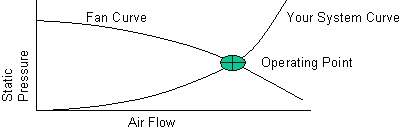Skip to content

# System Resistance

How the resistance to airflow in your system changes, as measured in pressure drop, for a given change in airflow.As flow through your system is increased, the pressure required increases by the square of that increase. 2 times the flow requires 4 times the pressure.

Once one point of flow and pressure is measured for your system, the system curve can be closely estimated.

The operating point is always on your system curve and always where it intersects the fan curve, equilibrium between the fan's ability to push air and your system's natural resistances to air flow.

The motion of air through electronic equipment can only be accomplished by the creation of a pressure drop across the piece of equipment, in the same manner that current can only be caused to flow through a resistance by the application of a voltage across it. This resistance to flow is defined by an equation of the form:

P = KpQn (eq.4)
Where P = pressure drop inches of water (inches w.g.).
Q = airflow in cu. ft/min (CFM).
K = a constant determined by the characteristics of the system.
n = a constant depending upon the type of flow.
p = density of air in lb. per cubic foot.
n will assume a value from 1 to 2 depending upon whether the flow in the system is completely laminar (n=1) or completely turbulent (n=2). For most electronic equipment, n will be found to be nearly equal to 2, and this value may be assumed for calculation purposes in absence of other data.

Due to the complex nature of the flow path typically found in electronic equipment, pressure drop calculations do not yield easily to the customary fluid flow equations, and most equipment designers find it best to resort to testing the equipment for its resistance characteristics.

When a fan is used to move air through electronic equipment, the flow through the systems is determined by the intersection of the performance curve and the resistance curve. At this point of operation, the pressure available from the fan to force air through the system is equal to the pressure required by the system for that flow.